Engineering ToolBox - Resources, Tools and Basic Information for Engineering and Design of Technical Applications!

# Air - Specific Heat vs. Temperature at Constant Pressure

## Online calculator with figures and tables showing specific heat (Cp and Cv) of dry air vs. temperature and pressure. SI and imperial units.

Specific heat (C) is the amount of heat required to change the temperature of a mass unit of a substance by one degree.

• Isobaric specific heat (Cp) is used for air in a constant pressure (ΔP = 0) system.
• Isochoric specific heat (Cv) is used for air in a constant-volume, (= isovolumetric or isometric) closed system.

Note! At normal atmospheric pressure of 1.013 bar - the specific heat of dry air - CP and CV - will vary with temperature. This may influence on the accuracy of air conditioning and industrial air handling process calculations. When calculating mass and volume flow of air in heated or cooled systems with high accuracy - the specific heat (= heat capacity) should be corrected according values in the figures and table below, or found by use of the calculator.

• For ordinary calculations - a value of specific heat cp = 1.0 kJ/kg K (equal to kJ/kg oC) or 0.24 Btu(IT)/lb °F - is normally accurate enough
• For higher accuracy - a value of Cp = 1.006 kJ/kg K (equal to kJ/kg oC) or 0.2403 Btu(IT)/lb °F  - is better

### Online Air Specific Heat Calculator

The calculator below can be used to estimate the specific heat of air at constant volum or constant pressure and at given temperature and pressure.
The output heat capacity is given as kJ/(kmol*K), kJ/(kg*K), kWh/(kg*K), kcal/(kg*K), Btu(IT)/(mol*°R) and Btu(IT)/(lbm*°R)

Temperature

Choose the actual unit of temperature: °C  °F  °R
Choose the actual pressure:  1 bara / 14.5 psia; 10 bara / 145 psia; 50 bara / 725 psia; 100 bara / 1450 psia;

as well as Specific heat  of Ammonia, Butane, Carbon dioxide, Carbon monoxide, Ethane, Ethanol, Ethylene, Hydrogen, Methane, Methanol, Nitrogen, Oxygen, Propane and Water.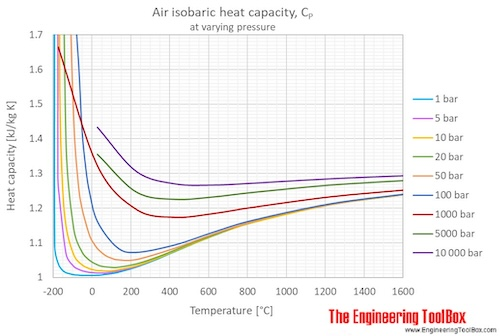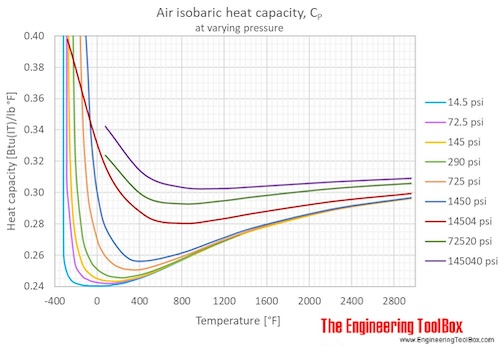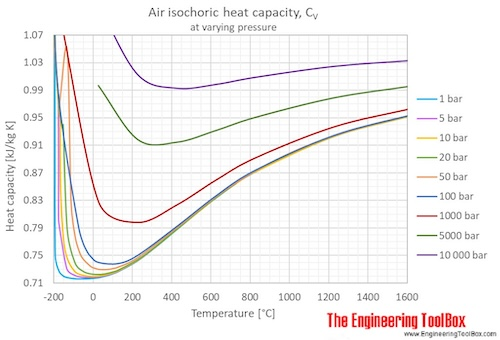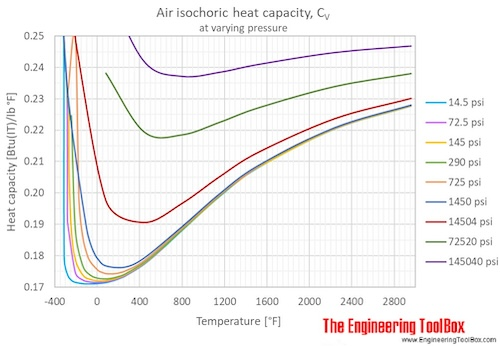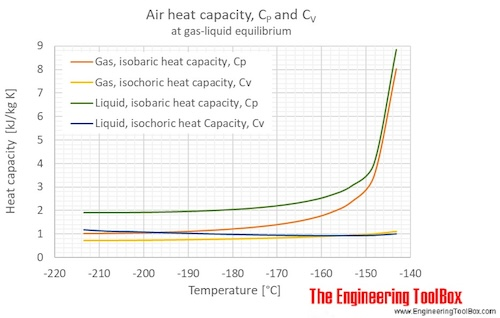Specific heat of air at 1 bar (=0.1 mPa = 14.5 psi):

For full table with Isobaric heat capacity - rotate the screen!

 Temperature Isochoric specific heat (Cv) Isobaric specific heat (Cp) Cp/Cv [K] [°C] [°F] [kJ/mol K] [kJ/kg K] [kWh/(kg K)] [kcal(IT)/(kg K)][Btu(IT)/lb °F] [kcal(IT)/(lb °F)] [kJ/mol K] [kJ/kg K] [(kW h)/(kg K)] [kcal(IT)/(kg K)][Btu(IT)/lb °F] [kcal(IT)/(lb °F)] [-] 60 -213 -352 0.03398 1.173 0.0003258 0.2802 0.2287 0.05506 1.901 0.000528 0.45405 0.37071 1.621 78.79 -194 -318 0.03044 1.051 0.0002919 0.2510 0.2050 0.05599 1.933 0.000537 0.46169 0.37695 1.839 81.61 -192 -313 0.02172 0.7500 0.0002083 0.1791 0.1463 0.03154 1.089 0.000303 0.26010 0.21237 1.452 100 -173 -280 0.02109 0.7280 0.0002022 0.1739 0.1420 0.03012 1.040 0.000289 0.24833 0.20276 1.428 120 -153 -244 0.02093 0.7225 0.0002007 0.1726 0.1406 0.02953 1.022 0.000283 0.24350 0.19930 1.415 140 -133 -208 0.02081 0.7184 0.0001996 0.1716 0.1401 0.02937 1.014 0.000282 0.24219 0.19774 1.411 160 -113 -172 0.02077 0.7172 0.0001992 0.1713 0.1399 0.02928 1.011 0.000281 0.24147 0.19716 1.410 180 -93.2 -136 0.02076 0.7166 0.0001991 0.1712 0.1397 0.02920 1.008 0.000280 0.24076 0.19657 1.407 200 -73.2 -99.7 0.02075 0.7163 0.0001990 0.1711 0.1397 0.02917 1.007 0.000280 0.24052 0.19638 1.406 220 -53.2 -63.7 0.02075 0.7163 0.0001990 0.1711 0.1397 0.02914 1.006 0.000279 0.24028 0.19618 1.404 240 -33.2 -27.7 0.02075 0.7164 0.0001990 0.1711 0.1397 0.02914 1.006 0.000279 0.24028 0.19618 1.404 260 -13.2 8.3 0.02076 0.7168 0.0001991 0.1712 0.1398 0.02914 1.006 0.000279 0.24028 0.19618 1.403 273.2 0.0 32.0 0.02077 0.7171 0.0001992 0.1713 0.1398 0.02914 1.006 0.000279 0.24028 0.19618 1.403 280 6.9 44.3 0.02078 0.7173 0.0001993 0.1713 0.1399 0.02914 1.006 0.000279 0.24028 0.19618 1.402 288.7 15.6 60.0 0.02078 0.7175 0.0001993 0.1714 0.1399 0.02914 1.006 0.000279 0.24030 0.19620 1.402 300 26.9 80.3 0.02080 0.7180 0.0001994 0.1715 0.1400 0.02915 1.006 0.000280 0.24036 0.19625 1.402 320 46.9 116 0.02083 0.7192 0.0001998 0.1718 0.1403 0.02917 1.007 0.000280 0.24052 0.19638 1.400 340 66.9 152 0.02087 0.7206 0.0002002 0.1721 0.1405 0.02923 1.009 0.000280 0.24100 0.19677 1.400 360 86.9 188 0.02092 0.7223 0.0002006 0.1725 0.1409 0.02926 1.010 0.000281 0.24123 0.19696 1.398 380 107 224 0.02098 0.7243 0.0002012 0.1730 0.1412 0.02931 1.012 0.000281 0.24171 0.19735 1.397 400 127 260 0.02105 0.7266 0.0002018 0.1735 0.1417 0.02937 1.014 0.000282 0.24219 0.19774 1.396 500 227 440 0.02150 0.7424 0.0002062 0.1773 0.1448 0.02983 1.030 0.000286 0.24597 0.20083 1.387 600 327 620 0.02213 0.7641 0.0002123 0.1825 0.1490 0.03044 1.051 0.000292 0.25103 0.20496 1.375 700 427 800 0.02282 0.7877 0.0002188 0.1881 0.1536 0.03114 1.075 0.000299 0.25675 0.20963 1.365 800 527 980 0.02351 0.8117 0.0002255 0.1939 0.1583 0.03183 1.099 0.000305 0.26249 0.21432 1.354 900 627 1160 0.02415 0.8338 0.0002316 0.1991 0.1626 0.03247 1.121 0.000311 0.26772 0.21858 1.344 1100 827 1520 0.02525 0.8716 0.0002421 0.2082 0.1700 0.03356 1.159 0.000322 0.27675 0.22596 1.329 1500 1227 2240 0.02673 0.9230 0.0002564 0.2204 0.1800 0.03505 1.210 0.000336 0.28901 0.23597 1.311 1900 1627 2960 0.02762 0.9535 0.0002649 0.2277 0.1859 0.03593 1.241 0.000345 0.29631 0.24193 1.301

Unit conversion:

Specific heat unit converter

british thermal unit(International table) = [Btu(IT)], degree celcius = [°C], degree fahrenheit = [°F], kelvin = [K], degree rankin = [°R], joule = [J], kilocalorie(International table) = [kcal(IT)], kilogram = [kg], kilojoule = [kJ], kilowatthour = [kWh], mole = [mol], pound =[lb]

K in the units can be replaced by °C, and vise versa. °R in the units can be replaced by °F, and vise versa.

• 1 Btu/(lb °F) = 1 Btu/(lb °R) = 1 kcal(IT)/(kg °C) = 1 kcal(IT)/(kg K) = 4186.8 J/(kg K) = 0.81647 kcal(IT)/(lb °F) = 1.163x10-3 kWh/(kg K)
• 1 J/(kg K) = 1 J/(kg °C) = 2.3885x10-4 kcal(IT)/(kg oC) = 2.3885x10-4 Btu/(lb °F) = 1.9501x10-4 kcal(IT)/(lb °F)
• 1 kcal(IT)/(kg °C) = 1 Btu/(lb °F) = 4186.8 J/(kg K) = 0.81647 kcal(IT)/(lb °F) = 1.163x10-3 kWh/(kg K)
• 1 kcal(IT)/(lb °F) = 1.2248 Btu/(lb °F) = 1.2248 kcal(IT)/(kg °C) = 5127.9 J/(kg K)
• 1 kJ/(kg K) = 1 kJ/(kg °C) = 1000 J/(kg K) = 1000 J/(kg °C) = 0.23885 kcal(IT)/(kg °C) = 0.23885 Btu/(lb °F) = 0.19501 kcal(IT)/(lb °F) = 2.7778x10-4 kWh/(kg K)
• 1 kWh/(kg K) = 0.85985 kcal(IT)/(kg °C) = 0.85985 Btu/(lb °F) = 3.6 kJ/(kg K)
• 1 mol of air = 28.96546 g

## Related Topics

• Air Psychrometrics - Moist and humid air - psychrometric charts, Mollier diagrams, air-condition temperatures and absolute and relative humidity and moisture content.
• Fluid Mechanics - The study of fluids - liquids and gases. Involving velocity, pressure, density and temperature as functions of space and time.
• Gases and Compressed Air - Air, LNG, LPG and other common gas properties, pipeline capacities, sizing of relief valves.
• Material Properties - Material properties of gases, fluids and solids - densities, specific heats, viscosities and more.

## Engineering ToolBox - SketchUp Extension - Online 3D modeling!

Add standard and customized parametric components - like flange beams, lumbers, piping, stairs and more - to your Sketchup model with the Engineering ToolBox - SketchUp Extension - enabled for use with the amazing, fun and free SketchUp Make and SketchUp Pro .Add the Engineering ToolBox extension to your SketchUp from the SketchUp Pro Sketchup Extension Warehouse!

Translate

## Privacy

We don't collect information from our users. Only emails and answers are saved in our archive. Cookies are only used in the browser to improve user experience.

Some of our calculators and applications let you save application data to your local computer. These applications will - due to browser restrictions - send data between your browser and our server. We don't save this data.

## Citation

• Engineering ToolBox, (2004). Air - Specific Heat vs. Temperature at Constant Pressure. [online] Available at: https://www.engineeringtoolbox.com/air-specific-heat-capacity-d_705.html [Accessed Day Mo. Year].

Modify access date.

. .

#### Scientific Online Calculator3 30